arma模型_arma模型matlab - CSDN

• 老师作业要求，实现ARMA和ARIMA模型的基本全过程和最后结果。 目录 所用的所有数据包 1，数据准备与预处理 （1）数据准备 （2）数据预处理 2，数据重采样 ...（1）ARMA模型构建 （2）模型好坏检验...
老师作业要求，实现ARMA和ARIMA模型的基本全过程和最后结果。

目录

所用的所有数据包

1，数据准备与预处理

（1）数据准备

（2）数据预处理

2，数据重采样

3，平稳性和非白噪声

（1）差分法实现

（2）平滑法处理

（4）非白噪声检验

4，时间序列定阶

（1）ACF和PACF定阶

5，构建模型和预测

（1）ARMA模型构建

（2）模型好坏检验

（3）ARIMA模型构建

总结

所用的所有数据包

import pandas as pd
import numpy as np
import seaborn as sns #热力图
import itertools
import datetime
import matplotlib.pyplot as plt
import statsmodels.api as sm
from statsmodels.stats.diagnostic import acorr_ljungbox #白噪声检验
from statsmodels.graphics.tsaplots import plot_acf,plot_pacf #画图定阶
from statsmodels.tsa.arima_model import ARIMA #模型
from statsmodels.tsa.arima_model import ARMA #模型
from statsmodels.stats.stattools import durbin_watson #DW检验
from statsmodels.graphics.api import qqplot #qq图

1，数据准备与预处理

（1）数据准备

自己做了点数据，做数据的代码如下：

def genertate_data():
index = pd.date_range(start='2018-1-1',end = '2018-9-1',freq='10T')
index = list(index)
data_list = []
for i in range(len(index)):
data_list.append(np.random.randn())
dataframe = pd.DataFrame({'time':index,'values':data_list})
dataframe.to_csv('C:\\Users\\happy\\Desktop\\old_data.csv',index=0)
print('the data is existting')


然后将它保存在了old_data.csv中，然后故意去将文件夹中的某些值，改成了-10000，弄成了异常值，（因为老师说尽可能显得步骤完整，最后分数才会高-，-所以我自己手动添加异常）

（2）数据预处理

这块的主要工作就是利用pandas里面的函数，去查看一下刚特殊操作后的数据。

def data_handle():
#print(data.describe()) #查看统计信息,发现最小值有-10000的异常数据
#print((data.isnull()).sum()) #查看是否存在缺失值
#print((data.duplicated()).sum()) #重复值
def change_zero(x):
if x == -10000:
return 0
else :
return x
data['values'] = data['values'].apply(lambda x: change_zero(x))

#利用均值填充缺失值
mean = data['values'].mean()
def change_mean(x):
if x == 0:
return mean
else:
return x
data['values'] = data['values'].apply(lambda x: change_mean(x))
#保存处理过的数据
data.to_csv('C:\\Users\\happy\\Desktop\\new_data.csv',index=0)
print('new data is existing')

2，数据重采样

为了得高分（-，-），做了很多个数据，然后一共有34992个数据，然后进行了一下重采样，数据以天进行重采样。

def Resampling(): #重采样
#将默认索引方式转换成时间索引
df['time'] = pd.to_datetime(df['time'])
df.set_index("time", inplace=True)

data = df['2018-1-1':'2018-8-1'] #取18-1-1到8-1做预测
test = df['2018-8-1':'2018-9-1']
data_train = data.resample('D').mean()  #以一天为时间间隔取均值,重采样
data_test = test.resample('D').mean()

return data_train,data_test

3，平稳性和非白噪声

由于ARMA和ARIMA需要时间序列满足平稳性和非白噪声的要求，所以要用查分法和平滑法（滚动平均和滚动标准差）来实现序列的平稳性操作。一般情况下，对时间序列进行一阶差分法就可以实现序列的平稳性，有时需要二阶查分。

（1）差分法实现


def stationarity(timeseries): #平稳性处理
#差分法(不平稳处理),保存成新的列,1阶差分,dropna() 删除缺失值
diff1 = timeseries.diff(1).dropna()
diff2 = diff1.diff(1) #在一阶查分基础上做二阶查分

diff1.plot(color = 'red',title='diff 1',figsize=(10,4))
diff2.plot(color = 'black',title='diff 2',figsize=(10,4))


可以看一下图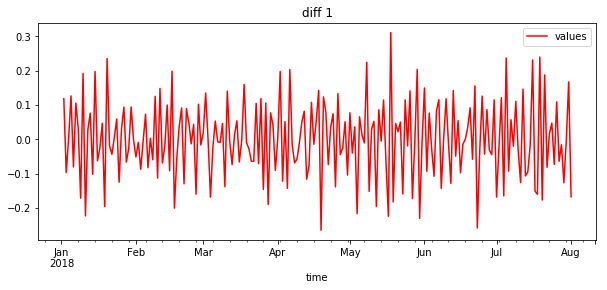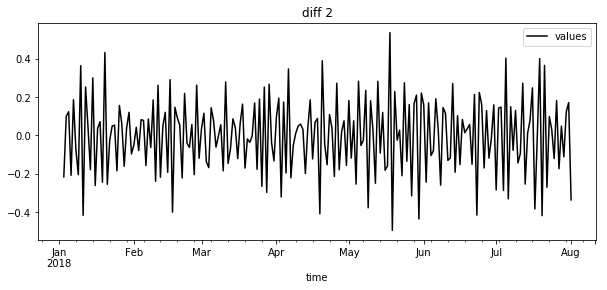一阶差分基本就满足了平稳性需要。

（2）平滑法处理

    #滚动平均（平滑法不平稳处理）
rolmean = timeseries.rolling(window=4,center = False).mean()
#滚动标准差
rolstd = timeseries.rolling(window=4,center = False).std()

rolmean.plot(color = 'yellow',title='Rolling Mean',figsize=(10,4))
rolstd.plot(color = 'blue',title='Rolling Std',figsize=(10,4))

处理结果如图所示。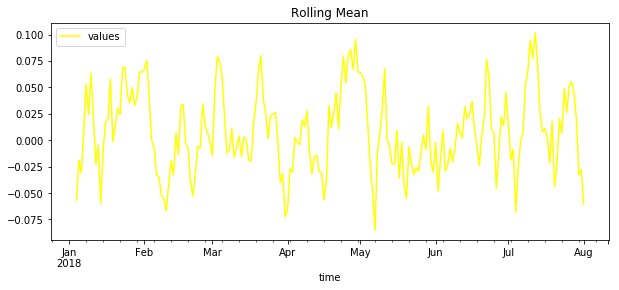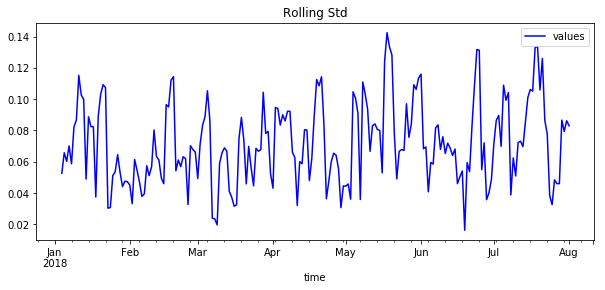可以看出，平滑法不太适合我造出来的数据，一般情况下，这种方法更适合带有周期性稳步上升的数据类型。

    #ADF检验
x = np.array(diff1['values'])
print (adftest) 

结果如下：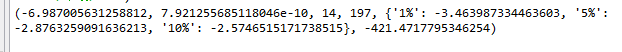如何确定该序列能否平稳呢？主要看：

（2）P-value是否非常接近0.本数据中，P-value 为 7.9e-10，接近0。

https://blog.csdn.net/weixin_42382211/article/details/81332431

（4）非白噪声检验

    #纯随机性检验（白噪声检验）
p_value = acorr_ljungbox(timeseries, lags=1)
print (p_value)

结果如图：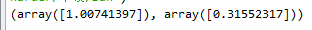统计量的P值小于显著性水平0.05，则可以以95%的置信水平拒绝原假设，认为序列为非白噪声序列（否则，接受原假设，认为序列为纯随机序列。）

由于P值为0.315远大于0.05所以接受原假设，认为时间序列是白噪声的，即是随机产生的序列，不具有时间上的相关性。（解释一下，由于老师没有给数据，所以只能硬着头皮，假设它是非白噪声的做）

4，时间序列定阶

定阶用到了ACF和PACF判断模型阶数、信息准则定阶（AIC、BIC、HQIC）、热力图定阶。

（1）ACF和PACF定阶

直接采用步骤3的一阶差分后的数据来进行定阶操作。


def determinate_order(timeseries):

#利用ACF和PACF判断模型阶数
plot_acf(timeseries,lags=40) #延迟数
plot_pacf(timeseries,lags=40)
plt.show()

结果如图所示：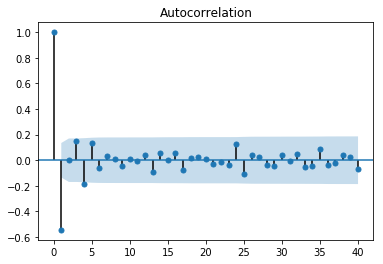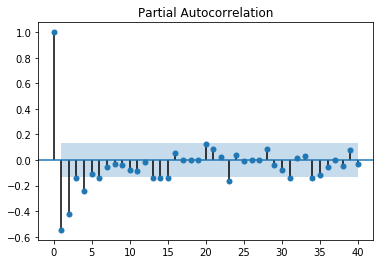上面分别是ACF和PACF的图，至于如何定阶不详细叙述了。一般是通过截尾和拖尾来确定阶数。目前还没有看到总结的比较好的文章。

（2）信息准则定阶

由于要通过ACF和PACF图来定阶，是一种看图的方法，因此可以计算AIC等值，来进行定阶。

    #信息准则定阶：AIC、BIC、HQIC
#AIC
AIC = sm.tsa.arma_order_select_ic(timeseries,\
max_ar=6,max_ma=4,ic='aic')['aic_min_order']
#BIC
BIC = sm.tsa.arma_order_select_ic(timeseries,max_ar=6,\
max_ma=4,ic='bic')['bic_min_order']
#HQIC
HQIC = sm.tsa.arma_order_select_ic(timeseries,max_ar=6,\
max_ma=4,ic='hqic')['hqic_min_order']
print('the AIC is{},\nthe BIC is{}\n the HQIC is{}'.format(AIC,BIC,HQIC))

一般都是一个一个运行，最好不要一起运行，结果出来的太慢了。

（3）热力图定阶

其实热力图定阶的方式和（2）信息准则定阶的方式类似，只是用热力图的方式呈现了。

    #设置遍历循环的初始条件，以热力图的形式展示，跟AIC定阶作用一样
p_min = 0
q_min = 0
p_max = 5
q_max = 5
d_min = 0
d_max = 5
# 创建Dataframe,以BIC准则
results_aic = pd.DataFrame(index=['AR{}'.format(i) \
for i in range(p_min,p_max+1)],\
columns=['MA{}'.format(i) for i in range(q_min,q_max+1)])
# itertools.product 返回p,q中的元素的笛卡尔积的元组
for p,d,q in itertools.product(range(p_min,p_max+1),\
range(d_min,d_max+1),range(q_min,q_max+1)):
if p==0 and q==0:
results_aic.loc['AR{}'.format(p), 'MA{}'.format(q)] = np.nan
continue
try:
model = sm.tsa.ARIMA(timeseries, order=(p, d, q))
results = model.fit()
#返回不同pq下的model的BIC值
results_aic.loc['AR{}'.format(p), 'MA{}'.format(q)] = results.aic
except:
continue
results_aic = results_aic[results_aic.columns].astype(float)
#print(results_bic)

fig, ax = plt.subplots(figsize=(10, 8))
ax = sns.heatmap(results_aic,
ax=ax,
annot=True, #将数字显示在热力图上
fmt='.2f',
)
ax.set_title('AIC')
plt.show() 

图如下所示：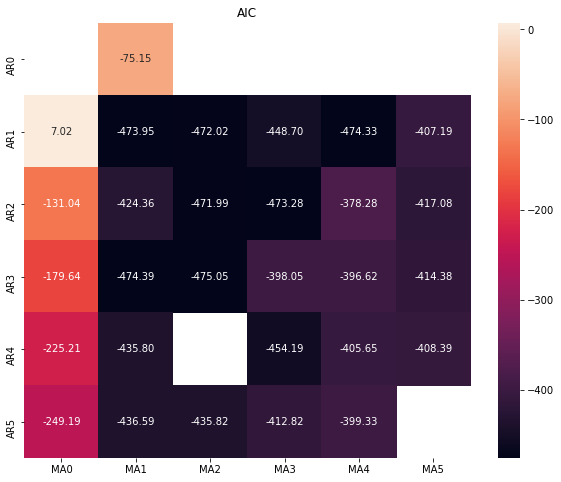黑色的位置最好，可以看出p，q取（1,1）（3,1）（1,4）都可以。一般情况下是越小越好。

热力图实现过程参考了下面这篇博客（找不见了=，=）如果有侵权，请告知，会删除博文。

5，构建模型和预测

（1）ARMA模型构建

def ARMA_model(train_D,train,test,order):
arma_model = ARMA(train,order) #ARMA模型
result = arma_model.fit()#激活模型
#print(result.summary()) #给出一份模型报告
############ in-sample ############
pred = result.predict()

#pred.plot()
#train.plot()
#print('标准差为{}'.format(mean_squared_error(train,pred)))

#残差
resid = result.resid
#利用QQ图检验残差是否满足正态分布
plt.figure(figsize=(12,8))
qqplot(resid,line='q',fit=True)
#利用D-W检验,检验残差的自相关性
print('D-W检验值为{}'.format(durbin_watson(resid.values)))

说明：train_D是重采样之后的数据，train是一阶查分后的，test是train样本外的数据，order给的是（1,1）通过定阶得到的。

以上都是通过参数传递进来的，没有在里面写。

预测过程有两种预测方式，一种是样本内的预测，一种是样本外的预测。意思就是，一个是根据train数据集的时间，在这个时间段内进行预测；样本外预测就是train的是2018-1-1到8-1的，但是要预测的是8-1到9-1的情况，是out-sample预测，一般情况下，out-sample是我们想要的，而不是样本内的预测。样本外的预测代码如下：

    pred_one = result.predict(start=len(train)-5,end = len(train)+30, \
dynamic=True)
#print(pred_one)
#print(len(test))
#print(pred_one[6:-1])
#pred_one.plot()
#test.plot()
print('标准差为{}'.format(mean_squared_error(test,pred_one[6:-1],sample_weight=None,\
multioutput='uniform_average'))) #标准差（均方差）

预测为样本内外是由dynamic参数决定的，特别注意：样本外的预测也要从样本内的某一个时间点开始才能进行预测。

在求解标准差的时候，要注意数据对齐。

（2）模型好坏检验

    #残差
resid = result.resid
#利用QQ图检验残差是否满足正态分布
plt.figure(figsize=(12,8))
qqplot(resid,line='q',fit=True)
#利用D-W检验,检验残差的自相关性
print('D-W检验值为{}'.format(durbin_watson(resid.values)))

用残差来检验模型的好坏，上述代码在创建模型里面面已经写过了，

qq图如下所示：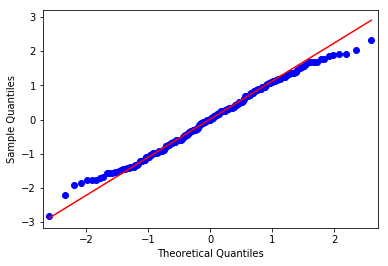通过qq图可以看出，残差基本满足了正态分布。

D-W检验结果为：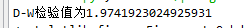当D-W检验值接近于2时，不存在自相关性，说明模型较好。

D-W检验如何数学说明，可以参考下面链接。

https://wenku.baidu.com/view/57224dcfcf84b9d528ea7aba.html

（3）ARIMA模型构建

创建模型的代码基本同上，只不过ARIMA有三个参数：p，d，q。其中p和q可以参考定阶的方法确定。d指的是用了多少阶差分，在我的模型中运用了一阶差分，因此d=1。

由于预测都是针对的差分法后的数据做的预测，但是真实数据并不是那样的，因此我还对差分后的数据进行还原操作。

看一下通过预测与实际真实值对比，模型到底是否很好。

def string_toDatetime(string):
return  datetime.datetime.strptime(string, "%Y-%m-%d %H:%M:%S")

def ARIMA_model(train_H,train,test):
arima_model = ARIMA(train,order =(1,1,1)) #ARIMA模型
result = arima_model.fit()
#print(result.summary()) #给出一份模型报告
########得到训练集的预测时间序列#########
pred = result.predict()

#######还原#########
##2018-8-1 00:00 到 2018-9-1 00:00 ###
#将差分后的序列还原,pred_restored为还原之后
idx = pd.date_range(string_toDatetime('2018-8-1 00:00:00'),periods=len(pred[4:20]),freq='D')
pred_list= []
for i in range(len(pred[4:20])):
pred_list.append(np.array(pred)[i+4])
pred_numpy = pd.Series(np.array(pred_list),index=idx)

pred_restored = pd.Series(np.array(train_H),\
index=[train_H.index]).append(pred_numpy).cumsum()
x1 = np.array(pred_restored)
x2 = np.array(train_H[5:22])
y = []
for i in range(len(pred_restored)):
y.append(i+1)
y = np.array(y)
fig1 = plt.figure(num=2, figsize=(10,4),dpi=80)
plt.plot(y,x1,color='blue')
plt.plot(y,x2,color='red')
plt.ylim(0,0.8)
plt.show()

代码里面对差分法后的进行还原，主要是下面这行代码：

    pred_restored = pd.Series(np.array(train_H),\
index=[train_H.index]).append(pred_numpy).cumsum()

主要用到了的是cumsum（）这个函数，这个函数作用不再赘述，自行百度吧。

总结

关于ARMA和ARIMA模型，从数据处理到最后建模实现，就完成了。

但是，里面其实有一个很大的问题，就是当数据不是平稳性的数据的时候，用到了差分法进行处理，用到了dropna()这个函数，这个函数的意思是去掉序列中nan（在这个了里面是0）。因此当序列中两列相邻值相等时，就会去掉前面那一列，因此处理后的数据可能不是按照每一天的数据分布的，但是预测出来的是每一天都存在的。

如果不加dropna（）这个函数的话，定阶那些都会报错（错误信息是存在nan值），但是模型不会报错。因此这块是一个存在的问题，还亟待处理，但是我看了很多的文章，对于这里好像没有过多深入的研究。

整篇博客都是代码实现的，具体的数学公式，自行百度吧~~

展开全文ARMA 时间序列预测
• 详细介绍ARMA模型 里面不仅介绍了该模型的实际用法也进行了举例分析arma
• ## ARMA模型概述

万次阅读 2018-05-25 10:18:36
ARMA模型在统计学角度来看，时间序列分析是统计学中的一个重要分支, 是基于随机过程理论和数理统计学的一种重要方法和应用研究领域. 时间序列按其统计特性可分为平稳性序列和非平稳性序列. 目前应用最多的是Box一...
  ARMA模型在统计学角度来看，时间序列分析是统计学中的一个重要分支, 是基于随机过程理论和数理统计学的一种重要方法和应用研究领域.  时间序列按其统计特性可分为平稳性序列和非平稳性序列. 目前应用最多的是Box一JenkinS 模型建模法, 它是由G.E.P.Box和英国统计学家G.M.JenkinS于1970年首次系统提出的.Box一JenkinS方法是一种较为完善的统计预测方法 , 他们的作用是为实际工作者提供了对时间序列进行分析、预测 , 以用对ARMA模型识别、估计和诊断的系统方法. 优点在于如果建立精确的模型后,并确定模型的系数之后,就可以根据有限的数据集对其发展进行预测 , 其中对于平稳性时间序列多采用ARMA模型 , 对于非平稳性时间序列模型常通过适当地变换 (如差分、取对数) 将它变为ARMA模型后再进行建模,这类模型Box一JenkinS称为ARI琳(求和自回归滑动平均模型) 。       参考链接：时序分析基础       一.  平稳时间序列模型       数据的预处理：              数据的预处理包括缺失值的补充、数据的平稳化及单位根检验.平稳性平稳性是时间序列分析中很重要的一个概念。一般的，我们认为一个时间序列是平稳的，如果它同时满足一下两个条件：1）均值函数是一个常数函数。2）自协方差函数只与时滞有关，与时间点无关。以上面两个时间序列为例。两个序列均满足条件1），因为标准正态分布白噪声和其形成的随机游走的均值函数都是值恒为0的常数函数。再来看条件2）。白噪声的自协方差函数可以表述为：可以看到只有在时滞为0时值为1，其它均为0，所以白噪声是一个平稳序列。而随机游走我们上面分析过，其自协方差为：很明显其自协方差依赖于时间点，所以是一个非平稳序列。后面可以看到，一般的时间序列分析往往针对平稳序列，对于非平稳序列会通过某些变换将其变为平稳的，例如，对于随机游走来说，其一阶差分序列是平稳的（显然其一阶差分是白噪声）。时序分析主要统计量       注意时间序列中的每一个元素都是一个普通的随机变量，如果忽略序列的时间性，那么我们面对的实际上是一个随机变量集合，所以从这个角度来说时间序列的统计分析与普通统计分析没有太大不同，相关的理论也是通用的。       对于随机变量集合来说，要完整描述其统计特性需要处理其多元联合分布，这是非常复杂的。所以实际我们往往做一些必要的简化假设，避免处理复杂的多元联合分布。现假设我们有随机时间序列下面先给出一些常用的统计量。后面会接着通过一些常见序列来举例说明各统计量如何计算。均值均值函数被定义为关于自变量t的函数：t的均值函数值表示在t时刻随机变量的期望。方差与均值类似，方差是t时刻序列元素的方差：自协方差自协方差是一个二元函数，其自变量为两个时间点，值是两个时间点上序列值的协方差：当t=s时，自协方差就是t时刻的方差。自相关系数自相关系数是两个时刻的值的相关系数：如果忽略元素来自时间序列这一事实，各统计量的意义实际上与普通的统计学中无异。因此这些统计量的一些性质也可以无缝推广到时间序列分析。例如期望的线性性质等等。如果有需要可以自行复习一下这些统计量的相关计算性质。后面的推导会主要集中于这几个统计量的计算。常见的随机时间序列常见的随机时间序列有：白噪音、布朗运动（随机游走）、白噪声       考虑一个时间序列，其中每一个元素为独立同分布变量，且均值为0。这种时间序列叫做白噪声。之所以叫这个名字，是因为对这种序列的频域分析表明其中平等的包含了各个频率，和物理中的白光类似。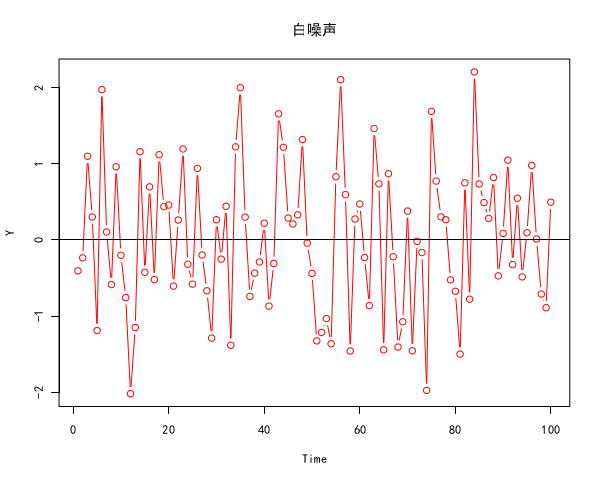没有模式即是白噪音的模式，所谓的白噪音即是随机性的完全体现，即是不能从白噪音中发现任何模式。以下是一段代码：[cpp] view plain copyY = ts(rnorm(100, mean=0, sd=1));  plot(Y, family="simhei", main="白噪声", type="b", col="red");  abline(h=0)       其中共100个元素，每个元素都独立服从标准正态分布。可以从图中看出白噪声基本是在均值附近较为平均的随机震荡。由于每个元素服从，所以均值，方差。又因为每个元素独立，所以对于任何，，。这些统计特征与对图像的直观观察基本一致。白噪声的重要之处在于很多其它的重要时间序列都可以通过它构造出来，这一点下文会看到。我们一般用e表示白噪声，将白噪声序列写作：布朗运动下面考虑这样一个时间序列，其在t时刻的值是前面白噪声序列的前t个值之和，设为标准正态分布产生的白噪声，则：布朗运动的模式在于其位置是连续曲线，但曲线的处处不可微。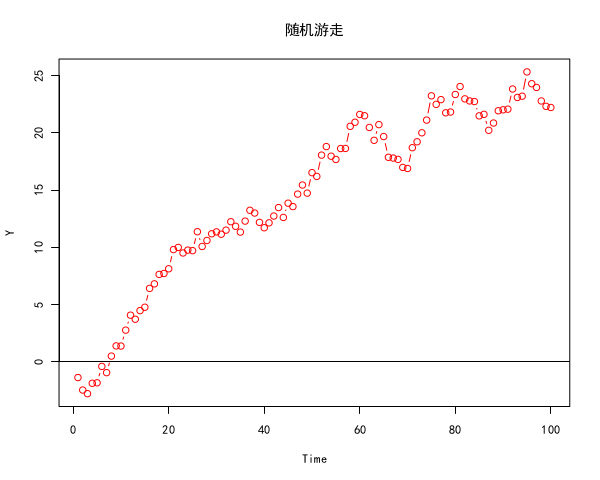[python] view plain copyY = ts(rnorm(100, mean=0, sd=1));  for (i in 2:length(Y)) {      Y[i] = Y[i] + Y[i-1];  }  plot(Y, family="simhei", main="随机游走", type="b", col="red");  abline(h=0)  可以看到随机游走比白噪声平滑很多，并且呈现出一些“趋势性”的感觉。下面分析其相关统计特征。均值：方差：对协方差的计算需要用到一个协方差性质：设t小于s，由于只有i=j时，所以：自协方差：自相关系数：从统计性质可以看到，随机游走的“趋势性”实际是个假象，因为其均值函数一直是白噪声的均值，不存在偏离的期望。但是方差与时间呈线性增长并且趋向于无穷大，这意味着只要时间够长，随机游走的序列值可以偏离均值任意远，但期望永远在均值处。物理与经济学中的很多现象都被看做是随机游走，例如分子的布朗运动，股票的价格走势等等。从协方差和相关系数看，如果起点t固定，则越接近的点相关性越大，例如，，。同时，起点不同，时滞相同自相关系数也不同，越往后同时滞自相关系数越大，例如，。实际上从纯数学角度可以将自相关系数看成一个二元函数，自变量是时间点t和时滞s-t。认识到这点很重要，因为它与时间序列分析中一个重要的概念——平稳性有着密切的关系。二、AR、MA、ARMA模型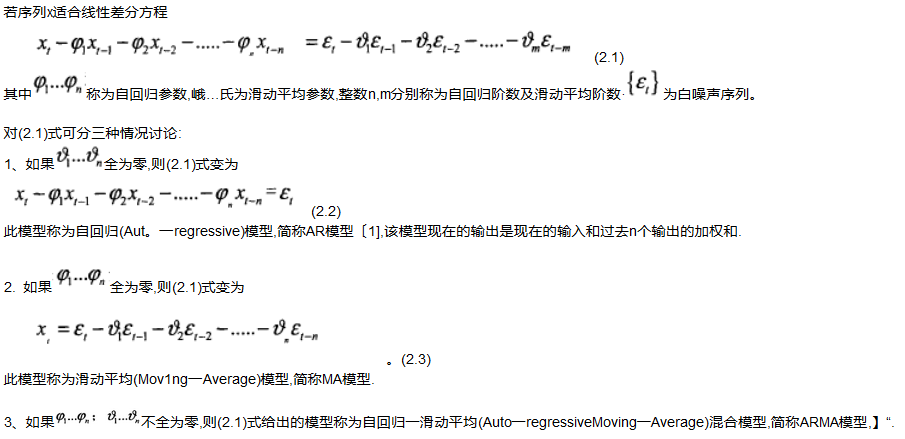4、AR、MA、ARMA认是平稳时间序列最主要的参数模型. AR模型的正则方程是一组线形方程 ,而MA和ARMA模型是非线性方程.Word分解定理告诉我们任何有限方差的ARMA或MA平移过程可以用可能是无限阶的AR模型表达;同样,任何ARMA或AR模型可以用可能是无限阶的撇模型表达.因此,如果在这三个模型中选一个与信号不匹配的模型,但只要模型的阶足够高,它能够比较好地逼近被建模的随机过程.三种模型中AR模型具有一系列好的性能,因此,是研究最多并获得广泛应用的一种模型。三、模型用于预测1.AR(n)模型预测       利用n之前的p个值对x(。)作预测,称之为“前向预测”,记为: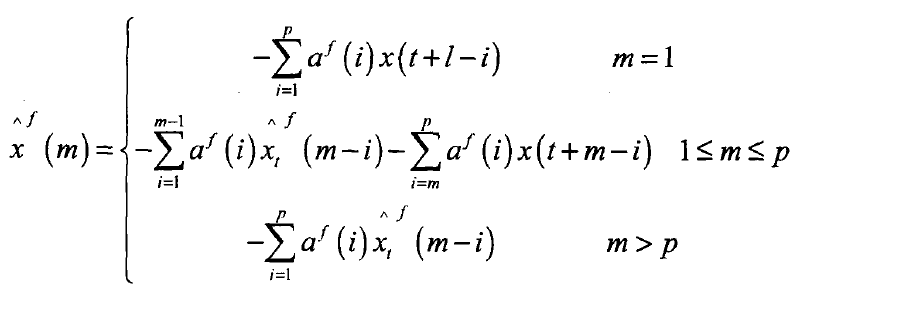上标f表示前向预测(forwardprediction)·凡(,)表示在t时刻m步前向预测。利用自相关法、Burg算法、协方差、改进的协方差法等方法得到模型的参数后,就可以进行前向预测,利用预测值递推可依次得到多步预测值2.MA(q)、ARMA(p,q)模型预测       MA(q)、ARMA(p,q)的外推预测一般都是将磁(q)、ARMA(p,q)模型转换为相应的高阶AR模型,再AR模型的预测公式进行外推预测.3.预测误差      预测误差公式为: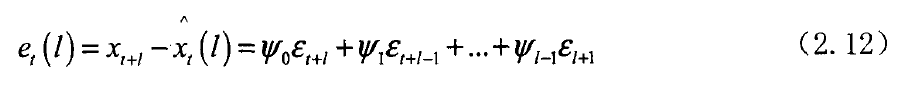线性最小方差预测的方差和预测步长l有关,而与预测的时间原点t无关.预测 步长l越大,预测误差的方差也越大,因而预测的准确度就会降低.所以一般不能用AR、MA和ARMA模型作为长期预测模型.
展开全文• ## 时间序列-ARMA模型

万次阅读 2019-02-19 19:34:02
ARMA(p,q) ... 注：ARMA(p,q)模型就是AR(p)和MA(q)模型的组合，更普遍的一类模型。  模型特征：  趋势性：无  相关性：有  随机性：有  ARMA(1,1)模型  一阶自回归移动平均模型  ...
ARMA(p,q)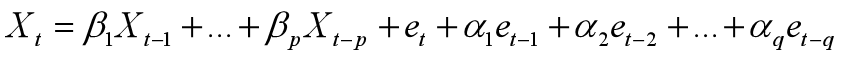注：ARMA(p,q)模型就是AR(p)和MA(q)模型的组合，更普遍的一类模型。

模型特征：

趋势性：无

相关性：有

随机性：有

ARMA(1,1)模型

一阶自回归移动平均模型

模型的表述 ：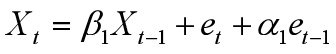该模型在t+1时的情形：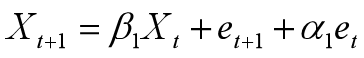ARMA(1,1)的序列相关性

通过查看自相关函数ACF和偏自相关函数PACF识别相关性

ACF呈指数下降趋势

PACF呈现指数下降趋势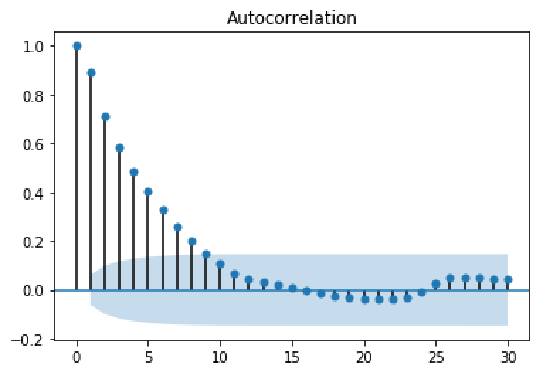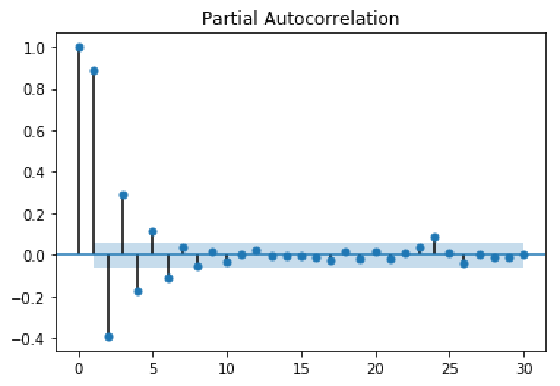如何识别一个ARMA的阶数p和q

由于ACF与PACF均呈现指数下降，判断阶数需要靠不断尝试

在模型的不同尝试中，通过选择AIC较小的为最优模型

AIC :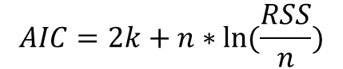,k为参数数量，RSS为残差平方和

ARIMA是在ARMA基础上发展而来的更加综合性的模型,体现为:

趋势性  序列相关性  随机性 

ARIMA是不平稳的时间序列，不能直接用ARMA建模

ARIMA 模型

ARIMA(p,d,q)的序列相关性识别：

差分后的ARIMA即为一个ARMA模型

利用ACF和PACF图像判定阶数 ARIMA(1,1,0)
展开全文ARMA 时间序列
• 数学建模中的ARMA模型和ARIMA模型的使用实例（含代码） 对于较少时间段的时间预测，因为数据量较少，所以直接使用神经网络是不现实的，这里用的比较多的是时间序列模型预测和灰色预测，这里介绍一下时间序列中ARMA...
数学建模中的ARMA模型和ARIMA模型的使用实例（含代码）

原文地址：http://blog.csdn.net/qq_34861102/article/details/77659399

对于较少时间段的时间预测，因为数据量较少，所以直接使用神经网络是不现实的，这里用的比较多的是时间序列模型预测和灰色预测，这里介绍一下时间序列中ARMA模型和ARIMA模型使用的实际例子

提供的一种误差检验： http://blog.csdn.net/qq_34861102/article/details/77930339

算法流程图：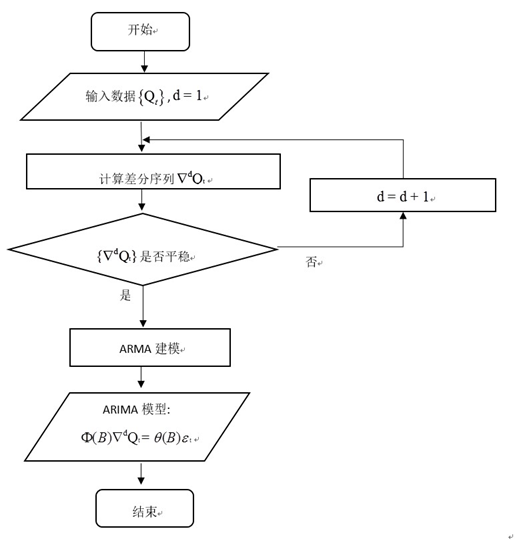1. 原始数据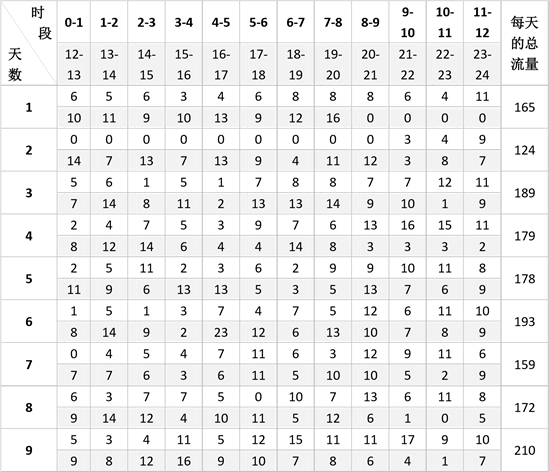这里是前九天的数据流量，一共有216个记录点

2. 寻找平稳时间序列

这里使用的是消除季节性和消除趋势以达到平稳时间序列的目的：

这里是实现差分后的图的表示：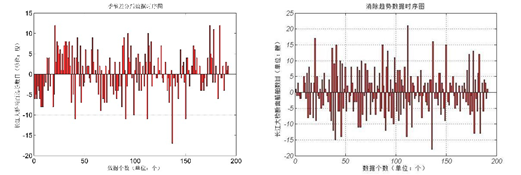这里我们使用的是消除季节性之后再消除趋势之后的图像作为输入的平稳时间序列

这里不是用Matlab中金融工具箱中的平稳性检验的函数，给大家推荐一个可以直接使用的平稳性函数的检验adftest  检验序列得到的结果是1可以证明是平稳时间序列。

3. 选定差分序列之后定阶层

这里确定了ARMA模型的输入的平稳时间序列之后选择定阶层。
这里直接使用双重for循环来找合适的阶层

这里选择查看不同的aic和bic来确定

当然可以使用自相关图和偏自相关图进行判断和检验
这里有Matlab自带的函数：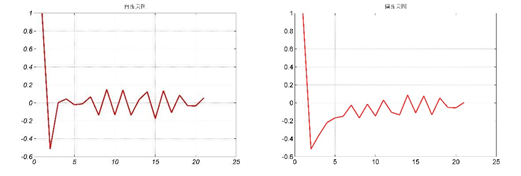4. 使用得到的ARMA模型之后反推ARIMA模型的参数估计

最后结果预测一天的示意图：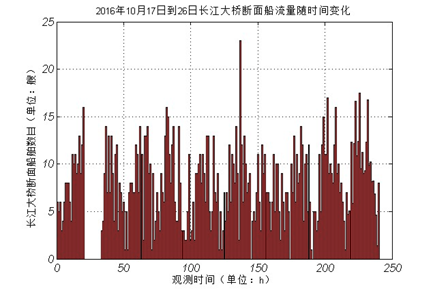和之前的数据做比较之后，可以看到实验的预测结果是拟合较好的

- 代码示例：

实现最佳阶层的选择：

s = 24; %周期是24
x = xixi';%初始数据的录入
n = 24; %预报的个数
m1 = length(x); %原始的数据的个数
for i = s+1:m1;
y(i-s) = x(i) - x(i-s);%进行周期差分变换
end
w = diff(y); %消除趋势性的差分运算
m2 = length(2);
for i = 0:6
for j = 0:6
if i == 0 & j == 0
continue
elseif i == 0
ToEstMd = arima('MALags',1:j,'Constant',0); %指定模型的结构
elseif j == 0
ToEstMd = arima('ARLags',1:i,'Constant',0); %指定模型的结构
else
ToEstMd = arima('ARLags',1:i,'MALags',1:j,'Constant',0); %指定模型的结构
end
k = k + 1;
R(k) = i;
M(k) = j;
[EstMd,EstParamCov,LogL,info] = estimate(ToEstMd,w');%模型拟合
numParams = sum(any(EstParamCov));%计算拟合参数的个数
[aic(k),bic(k)] = aicbic(LogL,numParams,m2);
end
end
fprintf('R,M,AIC,BIC的对应值如下\n%f');%显示计算结果
check  = [R',M',aic',bic']

开始用选定的阶层进行预测（p = 5  q = 2）：

x = xixi';

ToEstMd = arima('ARLags',1:5,'MALags',1:2,'Constant',0);%指定模型的结构
[EstMd,EstParamCov,LogL,info] = estimate(ToEstMd,w');%模型拟合
w_Forecast = forecast(EstMd,n,'Y0',w');
yhat = y(end) + cumsum(w_Forecast); %一阶差分的还原值
for j = 1:n
x(m1 + j) = yhat(j) + x(m1+j-s); %x的预测值
end
x(m1+1:end)
展开全文数学建模 实例 数据
• 本程序是一个完整的ARMA模型的识别、参数估计以及预测的代码，编写语言简单易懂，适合初学者
• 时间序列预测常用的方法有移动平均法，指数平均法，AR,MA,ARMA等，这类方法比较适用于小规模，单变量的预测，比如某门店的销量预测等，但是这些都要求序列是平稳的。 弱平稳序列 如果一个时间序列 xtx_txt​ 满足...
• %将训练数据和测试数据转为列向量 [data row data col] size data ; if data row<data col data data"; end dataiddata iddata data ; %参数如何定 model armax dataiddata [3 3] ; yp predict model data...
• 它们都统称为ARMA模型，而ARMA（p,q）模型的统计性质也是AR（p）与MA（q）模型的统计性质的有机组合。平稳系列建模假如某个观察值序列通过序列预处理可以判定为平稳非白噪声序列，就可以利用ARMA模型对序列建模。
• ## 关于ARMA模型的R语言实现

万次阅读 多人点赞 2019-06-22 15:06:38
本周首先更新的是用R来实现ARMA模型。 时间序列的模型，基本上都要建立在平稳的序列上，这里我们将来了解下ARIMA模型，以及其实现的R代码。 　ARMA（p,q)模型，全称移动平均自回归模型，它是由自回归（AR）部分和...R语言 ARMA模型
• ARMA自回归移动平均模型 金融时间序列分析：8. MA模型实例（Python） 金融时间序列分析：7. MA滑动平均模型 金融时间序列分析：6. AR模型实例 金融时间序列分析：5. AR模型实例（Python） 金融时间序列分析：4...python ARMA 自回归移动平均模型
• <br />最小二乘法（LS）要求系统的先验统计知识少...当利用高阶AR模型近似ARMA模型时，随着n的增大，计算量会较快增长。一般利用递推最小二乘法（RLS）公式估计参数（邓自立——《最有估计理论及其应用》p51）。算法
• <br />从ARMA到AR无穷 有限阶的ARMA模型可以等价为无穷阶的AR模型和MA模型，因而可以用高阶的AR模型和MA模型近似ARMA模型。 <br /> AR模型在建模方面明显比ARMA来的简单，而且它在最佳预测方面也较...
• ARMA 模型简介 ARMA 模型是研究时间序列的重要方法，由 AR 模型与 MA 模型混合而成。总的来说，AR 模型（自回归模型）是通过分析研究历史数据对当前数据的影响进行建模。MA 模型（移动平均模型）是用过去各个时期的...Python
• 它们都统称为ARMA模型，而ARMA（p,q）模型的统计性质也是AR（p）与MA（q）模型的统计性质的有机组合。平稳系列建模假如某个观察值序列通过序列预处理可以判定为平稳非白噪声序列，就可以利用ARMA模型对序列建模。时间序列 ARMA
• 一、时间序列与ARMA模型 自回归滑动平均模型（ARMA模型，Auto-Regression and Moving Average Model）是研究时间序列的重要方法，由自回归模型（AR模型）与滑动平均模型（MA模型）为基础“混合”而成，具有适用范围...时间序列 ARMA
• 推荐function [phi1,theta1,delta2]=arma... ARMA(1,1)模型的参数估计  %调用格式： [phi1,theta1,delta2]=arma110(Z) %Z为时间序列的向量 %Author: Ji Lin, 2007 W=Z-mean(Z); n=length(Z);K=15; r=ones(1,K);p=on
• 本文简单介绍了ARMA模型，包括其模型公式，统计特征，预测与分析…… ARMA简单来讲就是AR模型和MA模型的混合。 ARMA模型的提出是为了客服在表达数据时，经常出现高阶AR模型或MA模型，高阶模型由于其参数过多，复杂度...ARMA 自回归移动平均模型 时间序列分析
• 在统计学中，通常是建立一个线性模型来拟合该时间序列的趋势，其中，AR、MA、ARMA、ARIMA都是较为常用的模型。1、AR(Auto Regressive Model)自回归模型AR是线性时间序列分析模型中最简单的模型。通过自身前面部分的...ARMA 时间序列
...